# Relational Algebra Lecture 4 Relational Algebra Relational Algebra

• Slides: 54Relational Algebra Lecture 4Relational Algebra • • • Relational Algebra Extended Relational-Algebra-Operations Modification of the Database Views Operations on Bags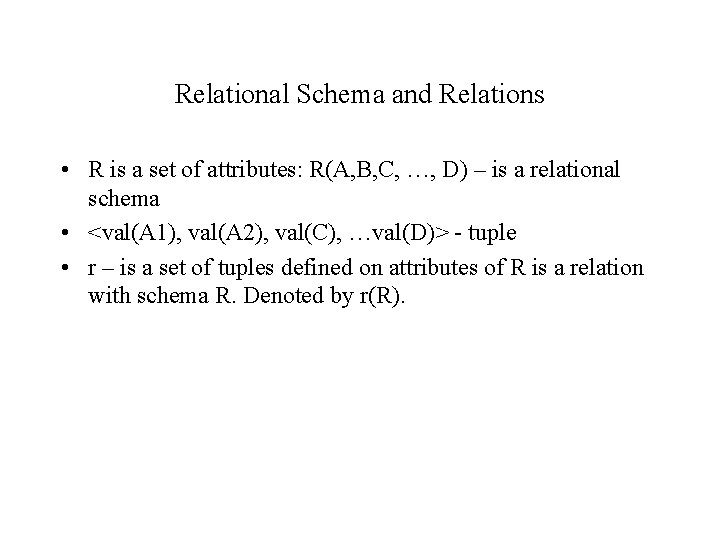Relational Schema and Relations • R is a set of attributes: R(A, B, C, …, D) – is a relational schema • <val(A 1), val(A 2), val(C), …val(D)> - tuple • r – is a set of tuples defined on attributes of R is a relation with schema R. Denoted by r(R).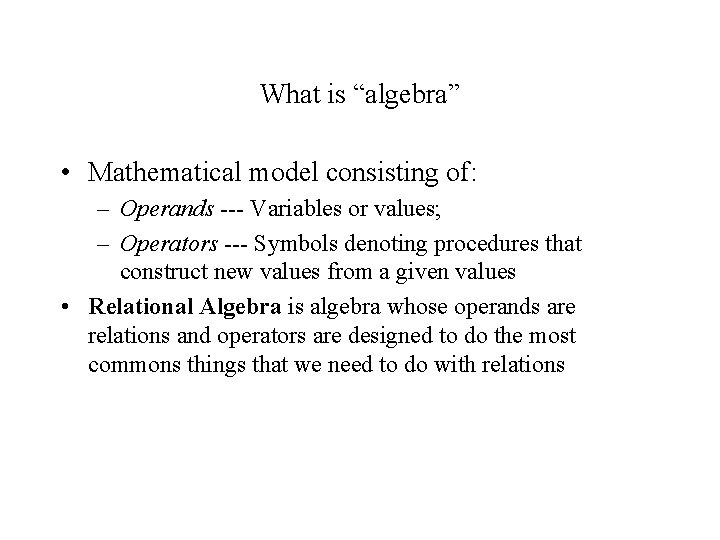What is “algebra” • Mathematical model consisting of: – Operands --- Variables or values; – Operators --- Symbols denoting procedures that construct new values from a given values • Relational Algebra is algebra whose operands are relations and operators are designed to do the most commons things that we need to do with relationsRelational Algebra • Six basic operators – Select – (r) – Project – P (r) – Union – r U s – set difference – r - s – Cartesian product – r X s – Rename – rename (r) • The operators take two or more relations as inputs and give a new relation as a result.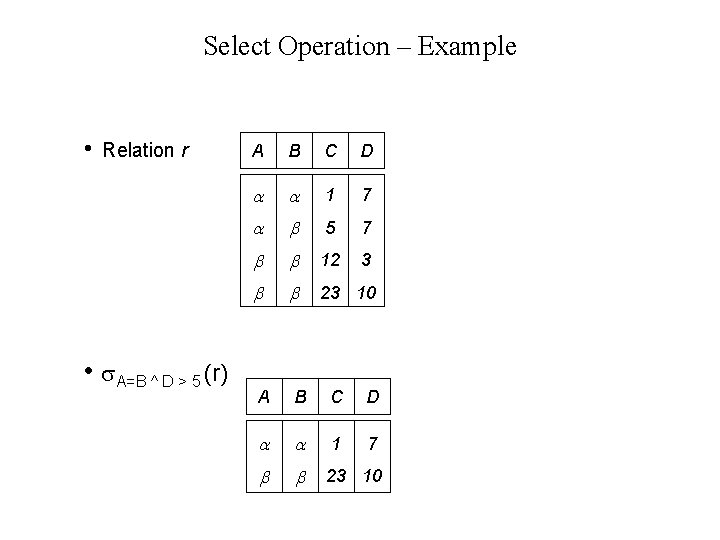Select Operation – Example • Relation r • A=B ^ D > 5 (r) A B C D 1 7 5 7 12 3 23 10 A B C D 1 7 23 10Select Operation • Notation: p(r) • p is called the selection predicate • Defined as: p(r) = {t | t r and p(t)} Where p is a formula in propositional calculus consisting of terms connected by : (and), (or), (not) Each term is one of: <attribute> op <attribute> or <constant> where op is one of: =, , >, . <. • Example of selection: branch-name=“Perryridge”(account)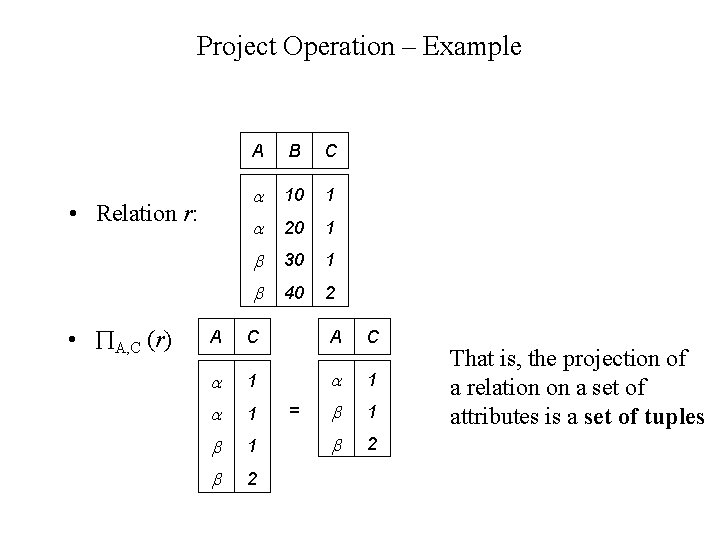Project Operation – Example • Relation r: • A, C (r) A B C 10 1 20 1 30 1 40 2 A C 1 1 1 2 2 = That is, the projection of a relation on a set of attributes is a set of tuplesProject Operation • Notation: A 1, A 2, …, Ak (r) where A 1, A 2 are attribute names and r is a relation. • The result is defined as the relation of k columns obtained by erasing the columns that are not listed • Duplicate rows removed from result, since relations are sets • E. g. to eliminate the branch-name attribute of account-number, balance (account)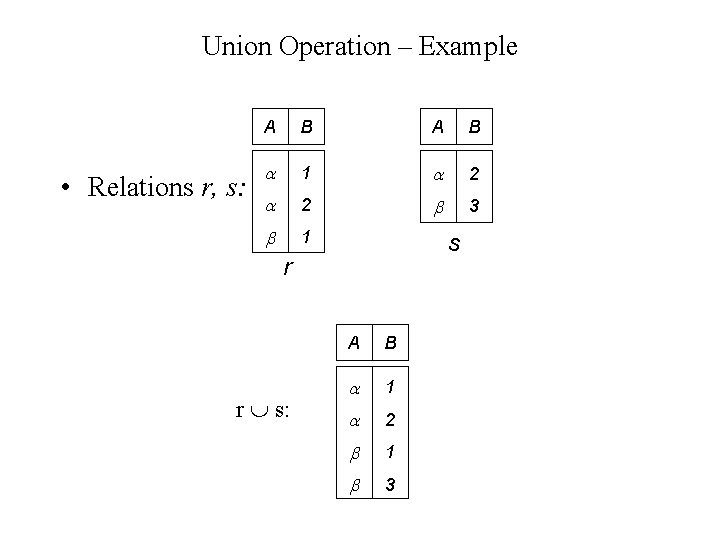Union Operation – Example • Relations r, s: A B 1 2 2 3 1 s r r s: A B 1 2 1 3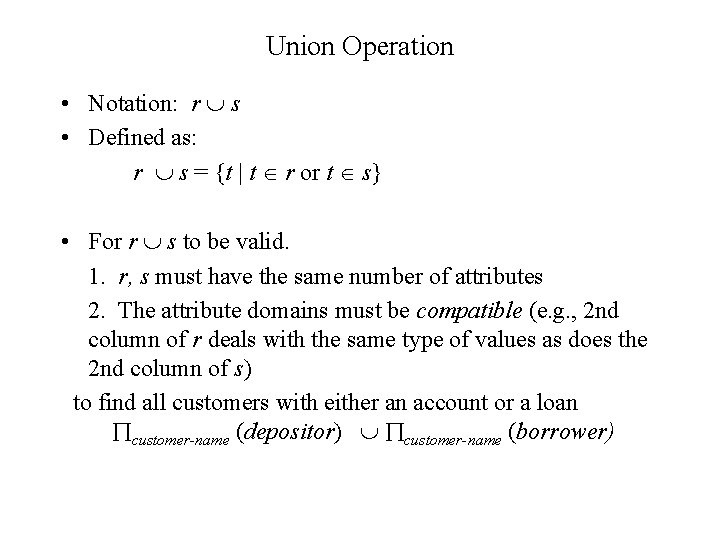Union Operation • Notation: r s • Defined as: r s = {t | t r or t s} • For r s to be valid. 1. r, s must have the same number of attributes 2. The attribute domains must be compatible (e. g. , 2 nd column of r deals with the same type of values as does the 2 nd column of s) to find all customers with either an account or a loan customer-name (depositor) customer-name (borrower)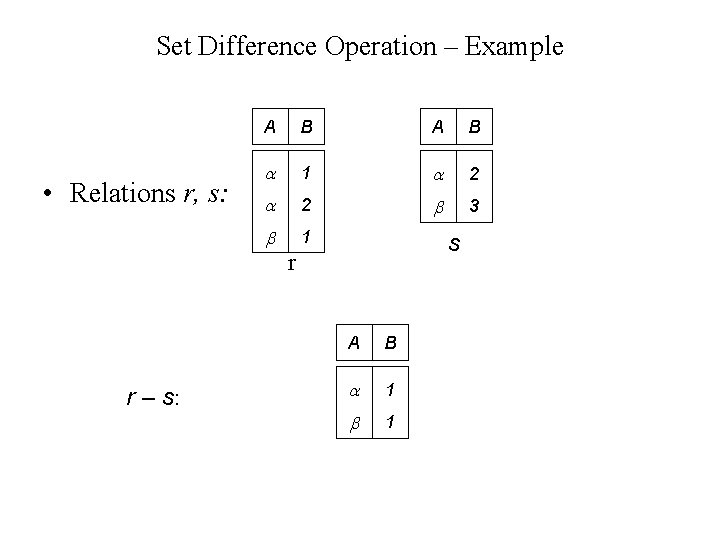Set Difference Operation – Example • Relations r, s: A B 1 2 2 3 1 s r r – s: A B 1 1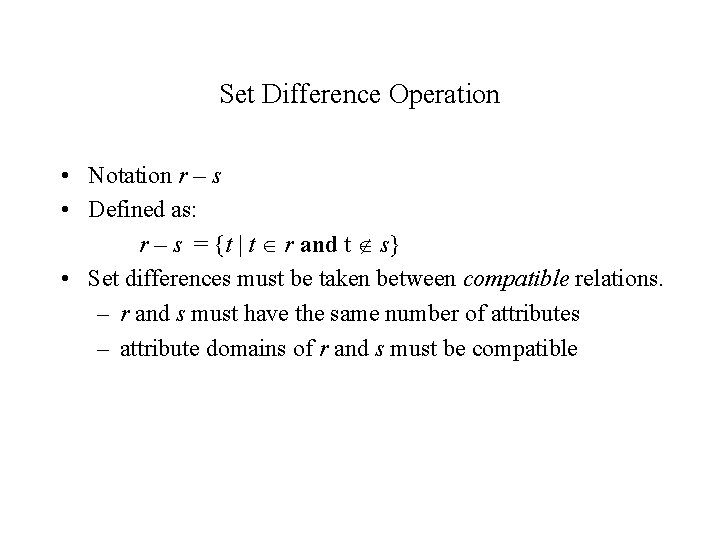Set Difference Operation • Notation r – s • Defined as: r – s = {t | t r and t s} • Set differences must be taken between compatible relations. – r and s must have the same number of attributes – attribute domains of r and s must be compatibleCartesian-Product Operation-Example Relations r, s: A B C D E 1 2 10 10 20 10 a a b b r s r x s: A B C D E 1 1 2 2 10 10 20 10 a a b b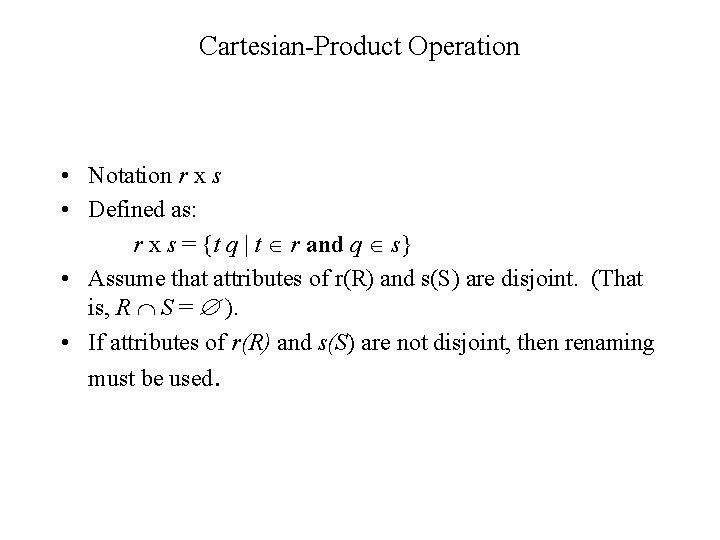Cartesian-Product Operation • Notation r x s • Defined as: r x s = {t q | t r and q s} • Assume that attributes of r(R) and s(S) are disjoint. (That is, R S = ). • If attributes of r(R) and s(S) are not disjoint, then renaming must be used.Composition of Operations • Can build expressions using multiple operations • Example: A=C(r x s) • rxs • A=C(r x s) A B C D E 1 1 2 2 10 10 20 10 a a b b A B C D E 1 2 2 10 20 a a bRename Operation • Allows us to name, and therefore to refer to, the results of relational-algebra expressions. • Allows us to refer to a relation by more than one name. Example: X (E) returns the expression E under the name X If a relational-algebra expression E has arity n, then (A 1, A 2, …, An) (E) xx E under the name X, and with returns the result of expression the attributes renamed to A 1, A 2, …. , An.Banking Example branch (branch-name, branch-city, assets) customer (customer-name, customer-street, customeronly) account (account-number, branch-name, balance) loan (loan-number, branch-name, amount) depositor (customer-name, account-number) borrower (customer-name, loan-number)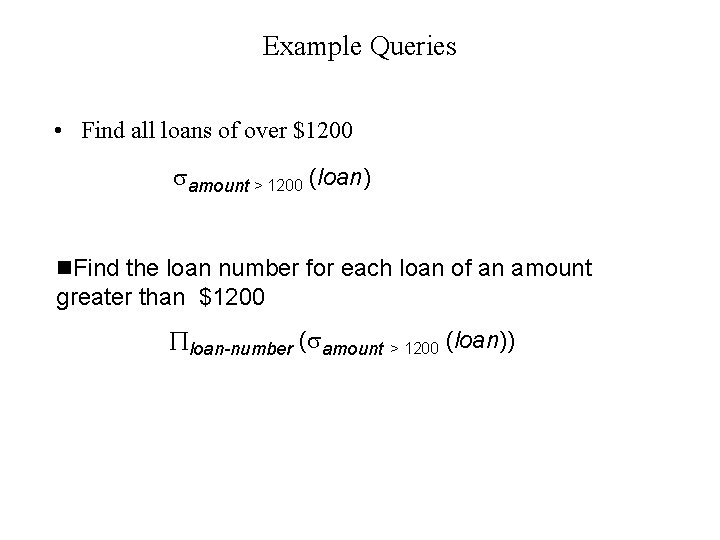Example Queries • Find all loans of over \$1200 amount > 1200 (loan) n. Find the loan number for each loan of an amount greater than \$1200 loan-number ( amount > 1200 (loan))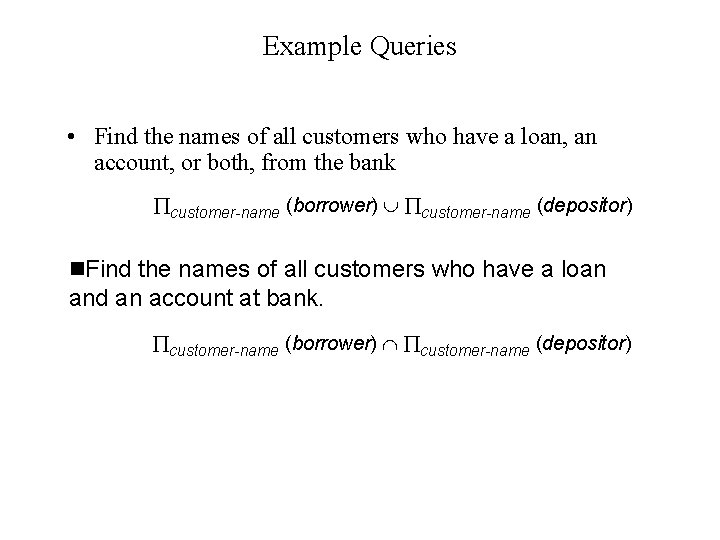Example Queries • Find the names of all customers who have a loan, an account, or both, from the bank customer-name (borrower) customer-name (depositor) n. Find the names of all customers who have a loan and an account at bank. customer-name (borrower) customer-name (depositor)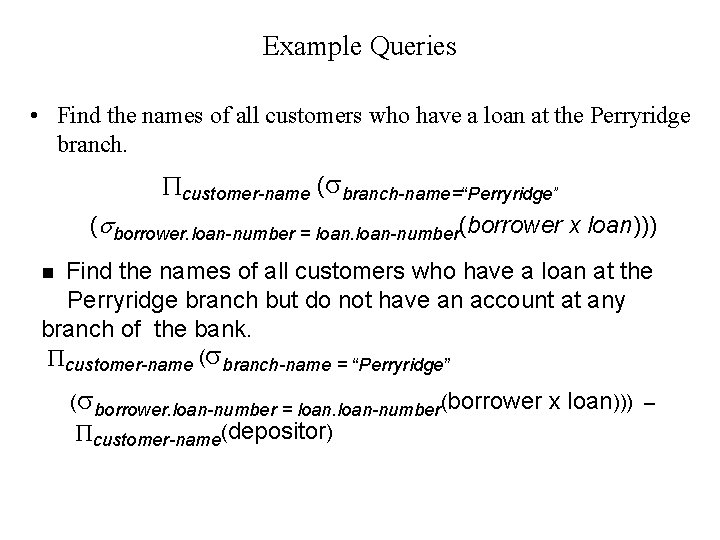Example Queries • Find the names of all customers who have a loan at the Perryridge branch. customer-name ( branch-name=“Perryridge” ( borrower. loan-number = loan-number(borrower x loan))) Find the names of all customers who have a loan at the Perryridge branch but do not have an account at any branch of the bank. n customer-name ( branch-name = “Perryridge” ( borrower. loan-number = loan-number(borrower customer-name(depositor) x loan))) –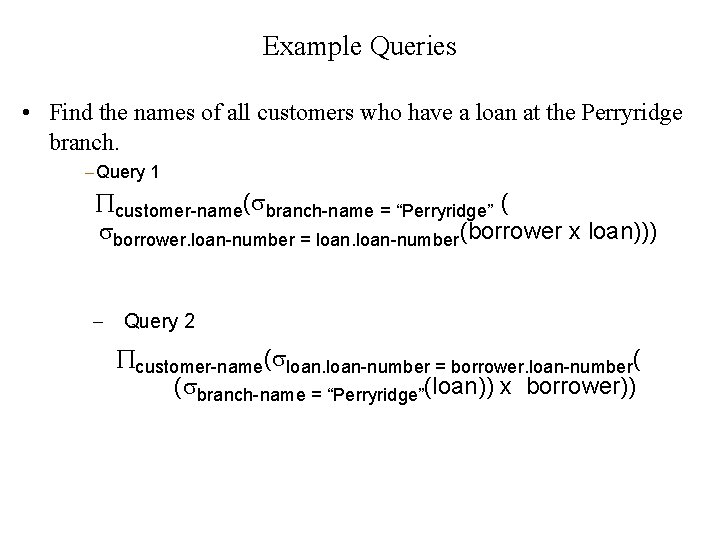Example Queries • Find the names of all customers who have a loan at the Perryridge branch. Query 1 customer-name( branch-name = “Perryridge” ( borrower. loan-number = loan-number(borrower x loan))) Query 2 customer-name( loan-number = borrower. loan-number( ( branch-name = “Perryridge”(loan)) x borrower))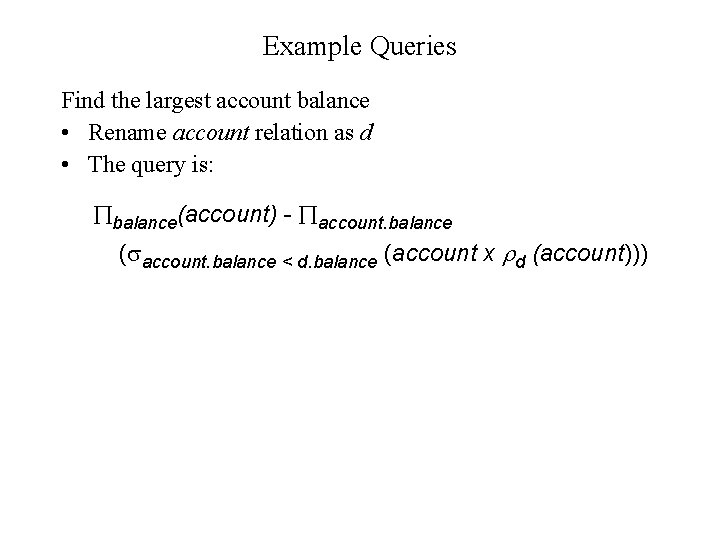Example Queries Find the largest account balance • Rename account relation as d • The query is: balance(account) - account. balance ( account. balance < d. balance (account x d (account)))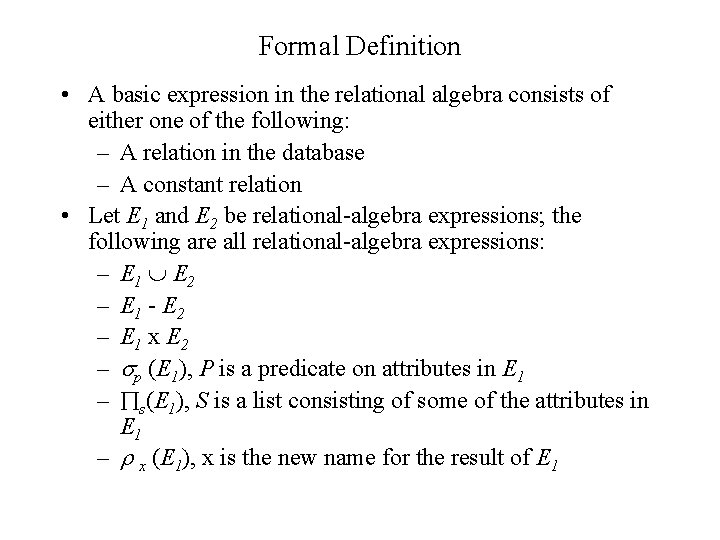Formal Definition • A basic expression in the relational algebra consists of either one of the following: – A relation in the database – A constant relation • Let E 1 and E 2 be relational-algebra expressions; the following are all relational-algebra expressions: – E 1 E 2 – E 1 - E 2 – E 1 x E 2 – p (E 1), P is a predicate on attributes in E 1 – s(E 1), S is a list consisting of some of the attributes in E 1 – x (E 1), x is the new name for the result of E 1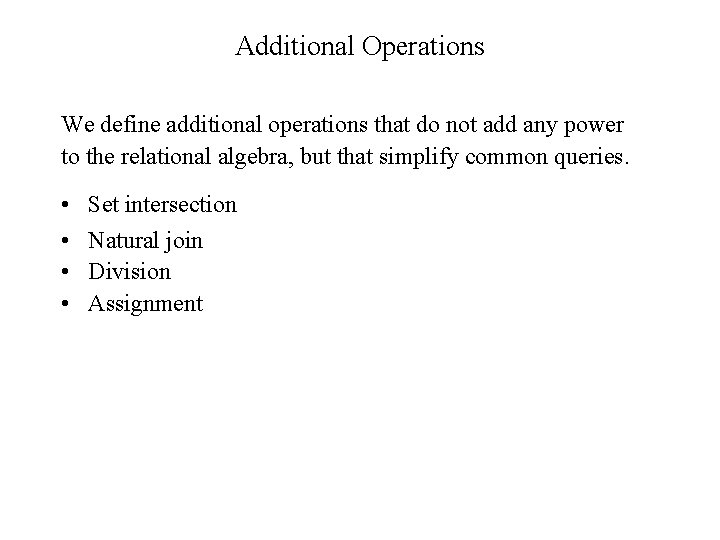Additional Operations We define additional operations that do not add any power to the relational algebra, but that simplify common queries. • • Set intersection Natural join Division AssignmentSet-Intersection Operation Notation: r s Defined as: r s ={ t | t r and t s } Assume: – r, s have the same arity – attributes of r and s are compatible • Note: r s = r - (r - s) • •Set-Intersection Operation - Example • Relation r, s: A B 1 2 1 r • r s A B 2 3 s A B 2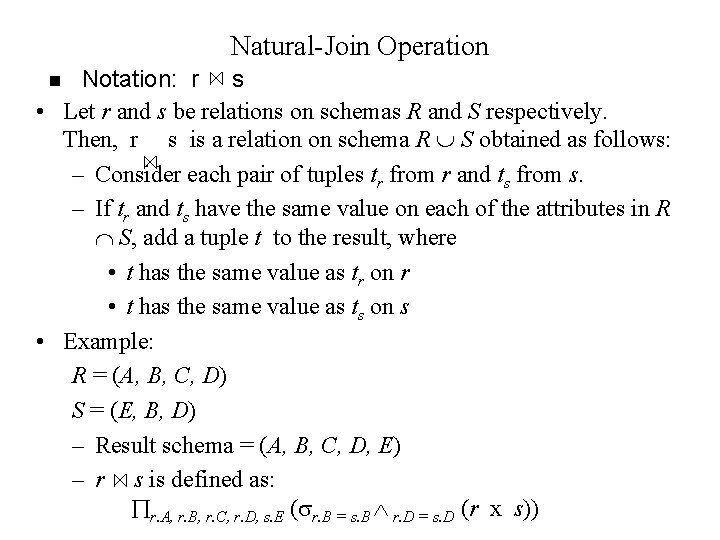Natural-Join Operation Notation: r s • Let r and s be relations on schemas R and S respectively. Then, r s is a relation on schema R S obtained as follows: – Consider each pair of tuples tr from r and ts from s. – If tr and ts have the same value on each of the attributes in R S, add a tuple t to the result, where • t has the same value as tr on r • t has the same value as ts on s • Example: R = (A, B, C, D) S = (E, B, D) – Result schema = (A, B, C, D, E) – r s is defined as: r. A, r. B, r. C, r. D, s. E ( r. B = s. B r. D = s. D (r x s)) nNatural Join Operation – Example • Relations r, s: A B C D B D E 1 2 4 1 2 a a b 1 3 1 2 3 a a a b b r r s s A B C D E 1 1 2 a a b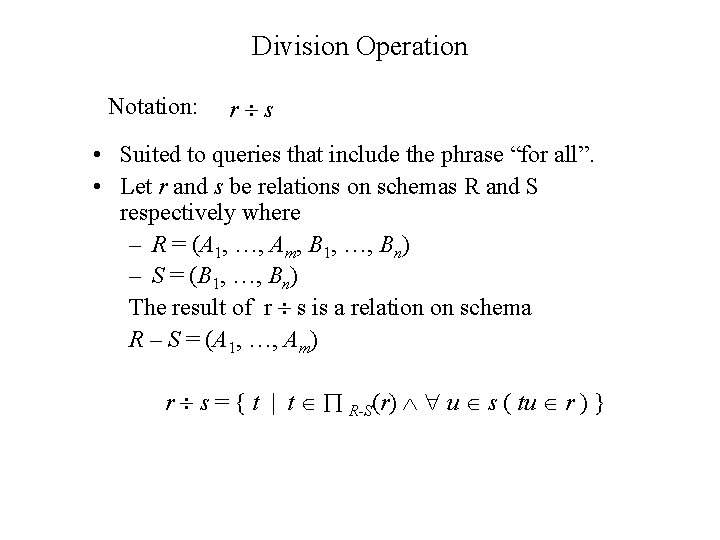Division Operation Notation: r s • Suited to queries that include the phrase “for all”. • Let r and s be relations on schemas R and S respectively where – R = (A 1, …, Am, B 1, …, Bn) – S = (B 1, …, Bn) The result of r s is a relation on schema R – S = (A 1, …, Am) r s = { t | t R-S(r) u s ( tu r ) }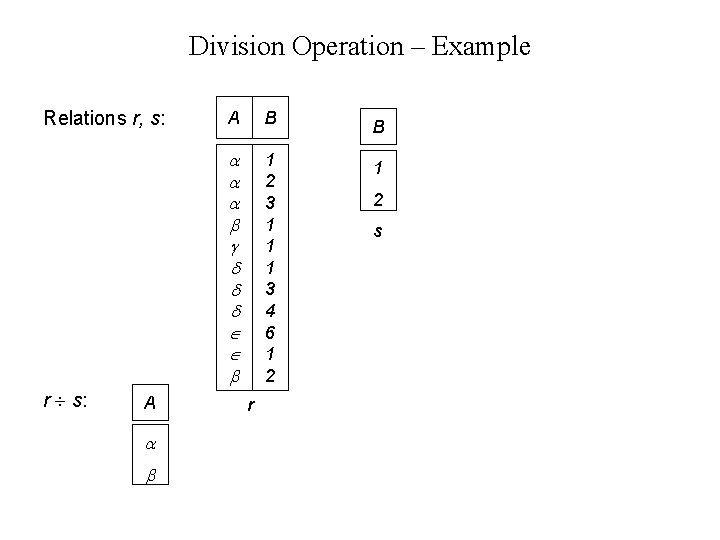Division Operation – Example Relations r, s: r s: A A B B 1 2 3 1 1 1 3 4 6 1 2 1 r 2 s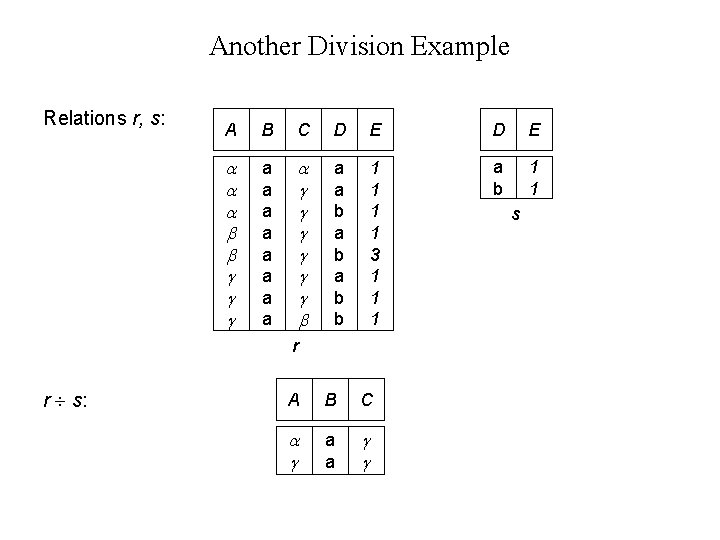Another Division Example Relations r, s: A B C D E a a a a a a b a b b 1 1 3 1 1 1 a b 1 1 r r s: A B C a a s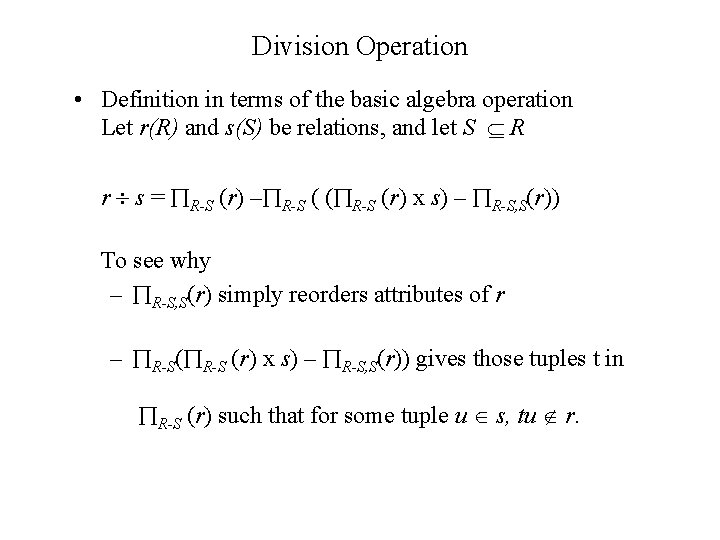Division Operation • Definition in terms of the basic algebra operation Let r(R) and s(S) be relations, and let S R r s = R-S (r) – R-S ( ( R-S (r) x s) – R-S, S(r)) To see why – R-S, S(r) simply reorders attributes of r – R-S( R-S (r) x s) – R-S, S(r)) gives those tuples t in R-S (r) such that for some tuple u s, tu r.Assignment Operation • The assignment operation ( ) provides a convenient way to express complex queries. – Write query as a sequential program consisting of • a series of assignments • followed by an expression whose value is displayed as a result of the query. – Assignment must always be made to a temporary relation variable. • Example: Write r s as temp 1 R-S (r) temp 2 R-S ((temp 1 x s) – R-S, S (r)) result = temp 1 – temp 2Example Queries • Find all customers who have an account from at least the “Downtown” and the Uptown” branches. Query 1 CN( BN=“Downtown”(depositor account)) CN( BN=“Uptown”(depositor account)) where CN denotes customer-name and BN denotes branch-name. Query 2 customer-name, branch-name (depositor account) temp(branch-name) ({(“Downtown”), (“Uptown”)})Example Queries • Find all customers who have an account at all branches located in Brooklyn city. customer-name, branch-name (depositor account) branch-name ( branch-city = “Brooklyn” (branch))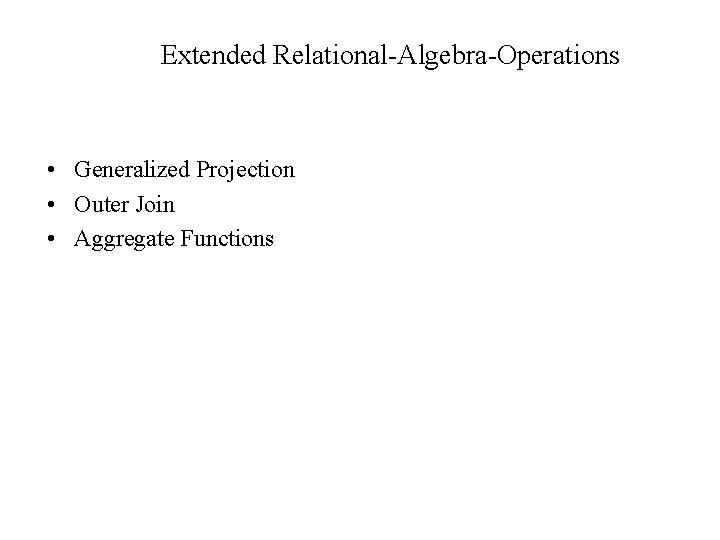Extended Relational-Algebra-Operations • Generalized Projection • Outer Join • Aggregate Functions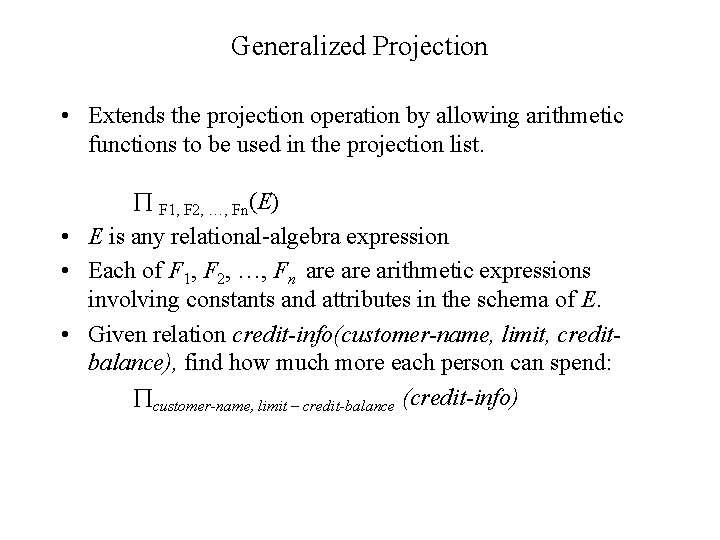Generalized Projection • Extends the projection operation by allowing arithmetic functions to be used in the projection list. F 1, F 2, …, Fn(E) • E is any relational-algebra expression • Each of F 1, F 2, …, Fn are arithmetic expressions involving constants and attributes in the schema of E. • Given relation credit-info(customer-name, limit, creditbalance), find how much more each person can spend: customer-name, limit – credit-balance (credit-info)Aggregate Functions and Operations • Aggregation function takes a collection of values and returns a single value as a result. avg: average value min: minimum value max: maximum value sum: sum of values count: number of values • Aggregate operation in relational algebra G 1, G 2, …, Gn g F 1( A 1), F 2( A 2), …, Fn( An) (E) – E is any relational-algebra expression – G 1, G 2 …, Gn is a list of attributes on which to group (can be empty) – Each Fi is an aggregate function – Each Ai is an attribute name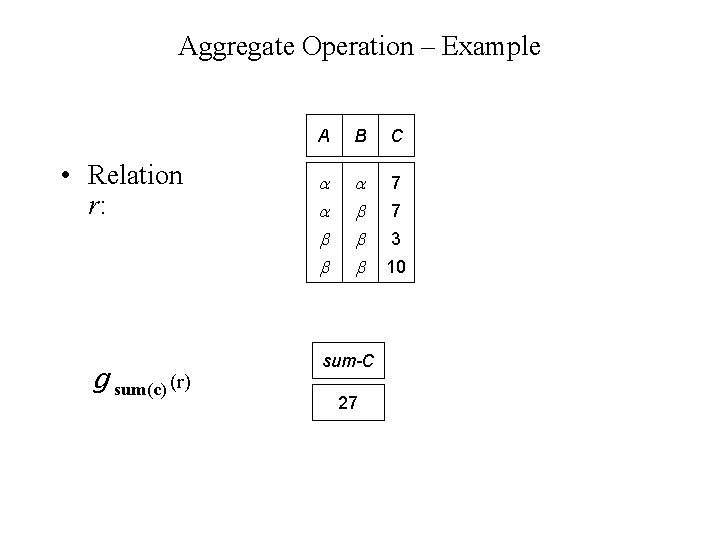Aggregate Operation – Example • Relation r: g sum(c) (r) A B C 7 sum-C 27 7 3 10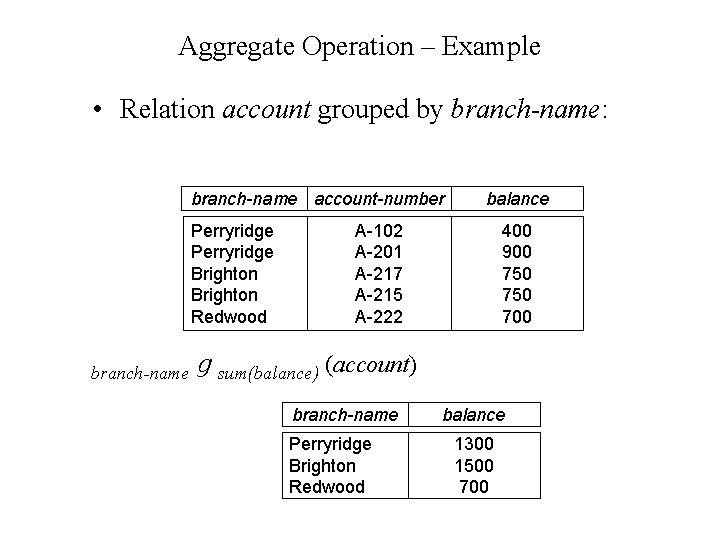Aggregate Operation – Example • Relation account grouped by branch-name: branch-name account-number Perryridge Brighton Redwood branch-name balance A-102 A-201 A-217 A-215 A-222 400 900 750 700 g sum(balance) (account) branch-name Perryridge Brighton Redwood balance 1300 1500 700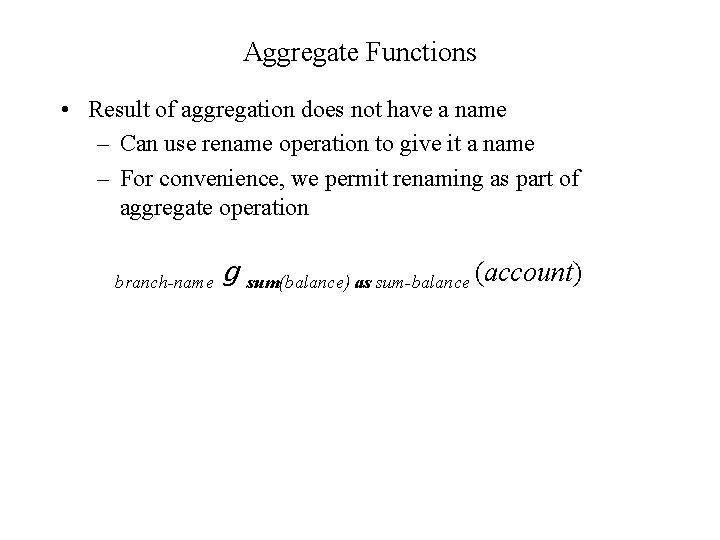Aggregate Functions • Result of aggregation does not have a name – Can use rename operation to give it a name – For convenience, we permit renaming as part of aggregate operation branch-name g sum(balance) as sum-balance (account)Outer Join – Example • Relation loan-number branch-name L-170 L-230 L-260 Downtown Redwood Perryridge amount 3000 4000 1700 n Relation borrower customer-name loan-number Jones Smith Hayes L-170 L-230 L-155Left Outer Join • Join loan Borrower loan-number branch-name L-170 L-230 Downtown Redwood amount customer-name 3000 4000 Jones Smith amount customer-name n Left Outer Join loan Borrower loan-number L-170 L-230 L-260 branch-name Downtown Redwood Perryridge 3000 4000 1700 Jones Smith null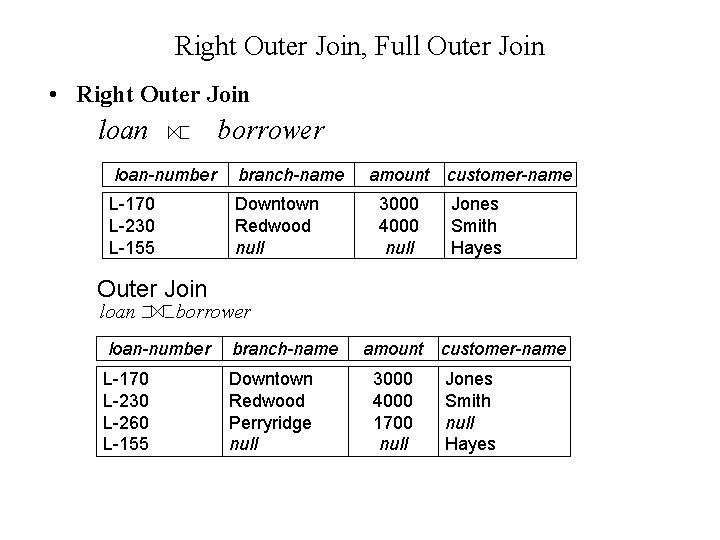Right Outer Join, Full Outer Join • Right Outer Join loan borrower loan-number branch-name L-170 L-230 L-155 Downtown Redwood null amount 3000 4000 null customer-name Jones Smith Hayes Outer Join loan borrower loan-number branch-name L-170 L-230 L-260 L-155 Downtown Redwood Perryridge null amount 3000 4000 1700 null customer-name Jones Smith null HayesNull Values • It is possible for tuples to have a null value, denoted by null, for some of their attributes • null signifies an unknown value or that a value does not exist. • The result of any arithmetic expression involving null is null. • Aggregate functions simply ignore null values • For duplicate elimination and grouping, null is treated like any other value, and two nulls are assumed to be the same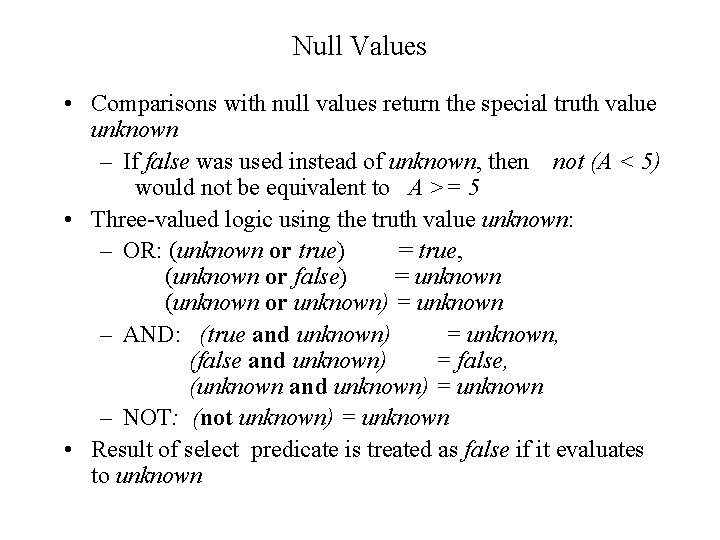Null Values • Comparisons with null values return the special truth value unknown – If false was used instead of unknown, then not (A < 5) would not be equivalent to A >= 5 • Three-valued logic using the truth value unknown: – OR: (unknown or true) = true, (unknown or false) = unknown (unknown or unknown) = unknown – AND: (true and unknown) = unknown, (false and unknown) = false, (unknown and unknown) = unknown – NOT: (not unknown) = unknown • Result of select predicate is treated as false if it evaluates to unknownExpression Trees Leaves are operands --- either variables standing for relations or particular relations Interior nodes are operators applied to their descendents customer-name, branch-name depositor accountRelational Algebra on Bags • A bag is like a set but it allows elements to be repeated in a set. • Example: {1, 2, 1, 3, 2, 5, 2} is a bag. • Difference between a bag and a list is that order is not important in a bag. • Example: {1, 2, 1, 3, 2, 5, 2} and {1, 1, 2, 3, 2, 2, 5} is the same bag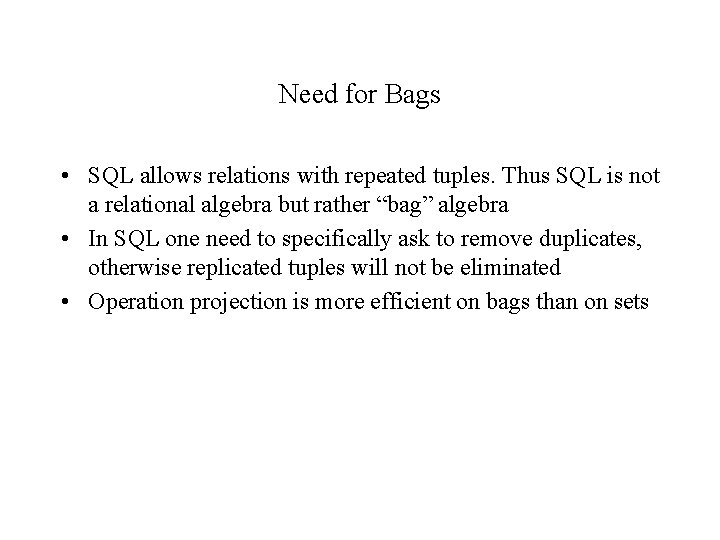Need for Bags • SQL allows relations with repeated tuples. Thus SQL is not a relational algebra but rather “bag” algebra • In SQL one need to specifically ask to remove duplicates, otherwise replicated tuples will not be eliminated • Operation projection is more efficient on bags than on setsOperations on Bags • Select applies to each tuple and no duplicates are eliminated • Project also applies to each tuple and duplicates are not eliminated. Example A B C 1 2 3 1 2 5 2 3 7 Projection on A, B A 1 1 2 B 2 2 3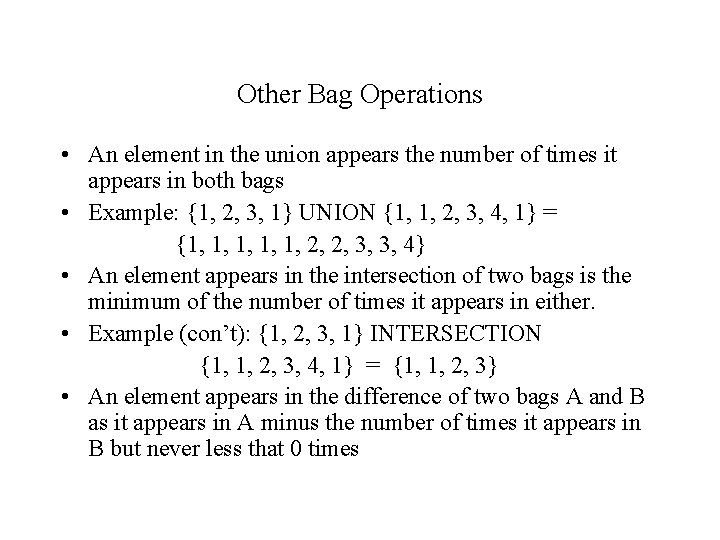Other Bag Operations • An element in the union appears the number of times it appears in both bags • Example: {1, 2, 3, 1} UNION {1, 1, 2, 3, 4, 1} = {1, 1, 1, 2, 2, 3, 3, 4} • An element appears in the intersection of two bags is the minimum of the number of times it appears in either. • Example (con’t): {1, 2, 3, 1} INTERSECTION {1, 1, 2, 3, 4, 1} = {1, 1, 2, 3} • An element appears in the difference of two bags A and B as it appears in A minus the number of times it appears in B but never less that 0 timesBag Laws • Not all laws for set operations are valid for bags: • Commutative law for union does hold for bags: R UNION S = S UNION R • However S union S = S for sets and it is not equal to S if S is a bag •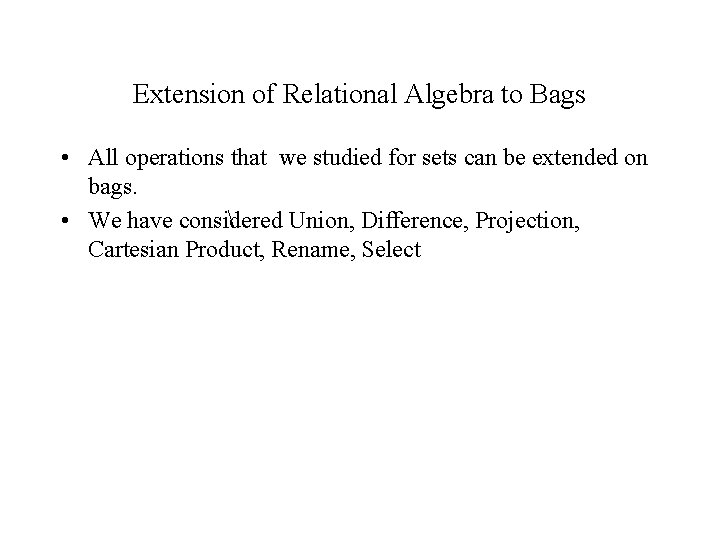Extension of Relational Algebra to Bags • All operations that we studied for sets can be extended on bags. • We have considered Union, Difference, Projection, Cartesian Product, Rename, Select